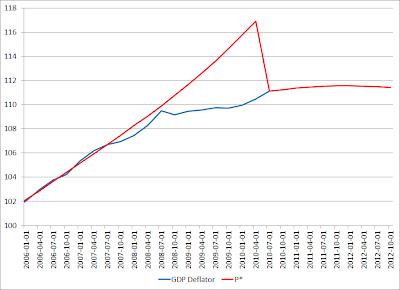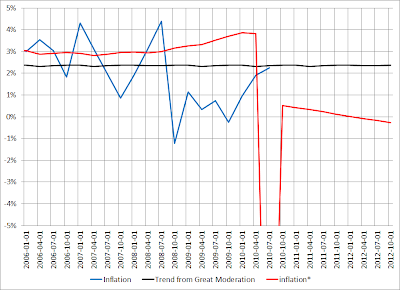Friday, November 19, 2010

Money Expenditure Targeting and the Productivity Norm

Advocates of the productivity norm argue that the growth rate of money expenditures should equal the growth rate of factor supplies. Growing productivity should result in a deflationary trend. Negative "supply shocks" should result in less deflation, and if extreme enough, stable prices or even inflation. Favorable supply shocks should result in greater deflation than usual. Assuming that the trend growth rate in factor supplies is 2 percent and factor productivity grows one percent, then a money expenditure rule consistent with the productivity norm should have a 2 percent growth rate of money expenditures. The trend deflation rate would be 1 percent.

Unfortunately, the CBO estimate of productive capacity suggests that the economy has suffering slow productivity growth recently. Further, money expenditures have fallen approximately 13 percent below the trend growth path of the Great Moderation. The price level has only fallen 2 percent below its trend growth path. The result is real expenditure and real output about 6.4 percent below the depressed estimate of productive capacity.

The price level as measured by the GDP deflator is 111 in the third quarter of 2010. The CBO estimate of potential income is \$14,167 billion in 2005 dollars. The level of money expenditures that would generate \$14,167 billion in real expenditures is \$15,740 billion.

Suppose a new 2 percent growth path starts now with the initial level being \$15,740 billion. The target for the third quarter of 2011 would be \$16,058 billion. Money expenditures would need to grow 10 percent to return to that growth path over next year.Money expenditures are initially at a level so that real expenditures equal the CBO estimate of potential income. Assuming that the CBO estimates are correct, then this initial price level will gently rise and then fall.

In the diagram below, the blue line shows the GDP implicit price deflator. The red lines shows what its value would be to keep real expenditures equal to the productive capacity of the economy, given the target for money expenditures. Up until the third quarter of 2010, this is assumed to be the 5 percent growth path of the Great Moderation and so the red line is showing an implicit price level target. What price level is consistent with keeping real expenditures equal to the CBO estimate of productive capacity, if money expenditures continued on the growth path of the Great Moderation. For the third quarter of 2010 and forward, what is shown is the price level consistent with real expenditures equal to the CBO estimate of the productive capacity of the economy, assuming money expenditures are on the adjusted growth path. There is a large drop in the implied target price level, so that it is equal to the actual price level starting in third quarter of 2010.The inflation rate is shown below. The black line shows the trend inflation rate from the Great Moderation. The blue line shows the actual inflation rate. The red line shows what the inflation rate would have been if money expenditures had remained on target and real expenditures remained equal to the CBO estimate of potential income. It is an implied target for the inflation rate. Up until the third quarter of 2010, this refelcts the 5 percent trend growth path of money expenditures from the Great Moderation. The large deflation shown is for the implied target, and since the price level is already well below that target, the result would by a shift from the actual inflation rate that happens to be almost exactly on the trend of the Great Moderation, to the new, much lower one.The sceneario described here involves a subtantial rapid increase in the growth of money expenditures--approximately 1o percent over the next year. The level of money expenditures at that time would be consistent with real expenditures equal to the CBO estimate of potential income. The "target" for the price level actually increases from 111.1 to 111.5. This slight inflation occurs because the CBO estimate of the growth path of productive capacity is only 1.6 percent over the period. Since the rule is based on the assumption that that factor supplies (like labor and capital) will rise 2 percent, productivity growth is negative. However, CBO estimates that productive capacity will begin to grow 2 percent starting at the first quarter of 2012 and rise above that, and so the rule will start to generate mild deflation after that point.

I still lean towards shifting to growth path of money expenditures that shifts to a 3 percent growth rate sometime in 2008. Rather than a productivity norm, I favor a stable trend price level, and accept that that the slowing of the growth rate of productivite capacity should be reflected in an adjustment to a higher price level. However, if one takes a more hardline anti-inflationary approach, it still looks like a very significant increase in the growth of money expenditures are appropriate over the next year.

1 comment:

1.We got to be very wise with all these things, as only then we will be able to perform well and achieve good results. I do it all nicely under OctaFX, as they are awesome by all measurements to do with been regulated plus a true ECN and that too an award winning one. I love the conditions with having lowest possible spreads at 0.1 pips for all major pairs while there is also 50% bonus on deposit which is use able.